--- title: "STATS 220" subtitle: "Data import`r emo::ji('arrow_down')`/export`r emo::ji('arrow_up')`" type: "lecture" date: "" output: xaringan::moon_reader: css: ["assets/remark.css"] lib_dir: libs nature: ratio: 16:9 highlightStyle: github highlightLines: true countIncrementalSlides: false --- ```{r initial, echo = FALSE, cache = FALSE, results = 'hide'} library(knitr) options(htmltools.dir.version = FALSE, tibble.width = 60, tibble.print_min = 6) opts_chunk\$set( echo = TRUE, warning = FALSE, message = FALSE, comment = "#>", fig.path = 'figure/', cache.path = 'cache/', cache = TRUE, fig.align = 'center', fig.width = 12, fig.height = 8.5, fig.show = 'hold', dpi = 120 ) ``` ```{r xaringan-panelset, echo = FALSE} xaringanExtra::use_panelset() ``` ```{r external, include = FALSE, cache = FALSE} read_chunk('R/02-import-export.R') ``` ## Atomic vector (1d) .center[] ```{r vector} ``` .footnote[image credit: Jenny Bryan] ??? * an ensemble of scalars -> vectors --- ## 1d `r emo::ji("arrow_right")` 2d .pull-left[

.center[] ] .pull-right[ ```{r tibbles} ``` ] .footnote[image credit: Jenny Bryan] ??? * an ensemble of vectors -> rect data/tabular data, like spreadsheet --- class: inverse middle ## Beyond 1d vectors ### 1. Lists ### 2. Matrices and arrays ### 3. Data frames and tibbles ??? * Common data strs beyond 1d * start with the most flex one * briefly talk about mat * focus on data frames, more specifically tibbles --- .left-column[ ## data strs ### - lists ] .right-column[ An object contains elements of **different data types**. ```{r lists} ``` ] ??? * to create a list using `list()` * put 4 atomic vectors inside my lst * a list of 4 elements, or length of 4 --- .left-column[ ## data strs ### - lists ] .right-column[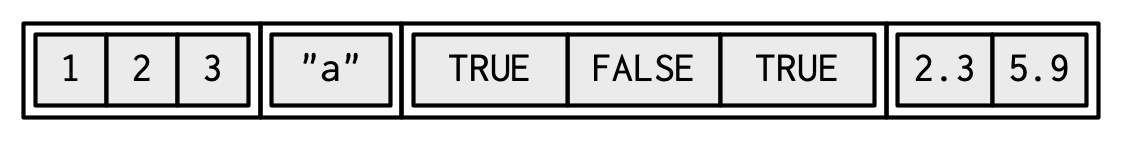.pull-left[ ## data type ```{r lists-type} ``` ## data class ```{r lists-cls} ``` ] .pull-right[ ## data structure ```{r lists-str, results = "hold"} ``` ] ] ??? * vis rep: a container, 4 items inside * primitive: original, cannot be modified * class: type + attrs, can be modified * rstudio values uses `str()` --- .left-column[ ## data strs ### - lists ] .right-column[ .pull-left[ ```{r ref.label = "lists", echo = 2} ``` ] .pull-right[ .center[] ] ] --- .left-column[ ## data strs ### - lists ] .right-column[ A list can contain other lists, i.e. **recursive** ```{r lists-rec} ``` ] ??? * most flex: put a list into a list * a named list --- .left-column[ ## data strs ### - lists ] .right-column[ .pull-left[ Test for a list ```{r is-list} ``` ] .pull-right[ Coerce to a list ```{r as-list} ``` ] ] ??? * to test if an object is one type, funs prefixed `is` * to coerce/convert from one type to another type, funs prefixed with `as` * from a vector of integers to a list --- .left-column[ ## data strs ### - lists ] .right-column[ .pull-left[ Subset by `[]` ```{r lst-sub} ``` ] .pull-right[ Subset by `[[]]` ```{r lst-sub2} ``` ] .center[![](img/pepper.png)] .footnote[image credit: Hadley Wickham] ] --- .left-column[ ## data strs ### - lists ### - matrices ] .right-column[ 2D structure of homogeneous data types * `matrix()` to construct a matrix ```{r matrix} ``` * `as.matrix()` to coerce to a matrix * `is.matrix()` to test for a matrix ] ??? * we don't deal with matrix in 220, matrix for computational stats. --- .left-column[ ## data strs ### - lists ### - matrices ] .right-column[ **array**: more than 2D matrix ```{r array} ``` ] --- .left-column[ ## data strs ### - lists ### - matrices ### - tibbles ] .right-column[ A data frame is a **named list** of vectors of the **same length**. ```{r data-frame} ``` ] --- .left-column[ ## data strs ### - lists ### - matrices ### - tibbles ] .right-column[ The underlying data type is a list. ```{r df-type} ``` .pull-left[ .center[data class] ```{r df-cls} ``` ] .pull-right[ .center[data attributes (meta info)] ```{r df-attrs} ``` ] ] ??? * `data.frame` represents tabular data in R * attributes: colnames and rownames --- .left-column[ ## data strs ### - lists ### - matrices ### - tibbles ] .right-column[ A tibble is a **modern reimagining** of the data frame. ```{r ref.label = "tibbles"} ``` * `as_tibble()` to coerce to a tibble * `is_tibble()` to test for a tibble ] ??? * why we call it `tibble` --- .left-column[ ## data strs ### - lists ### - matrices ### - tibbles ] .right-column[ .center[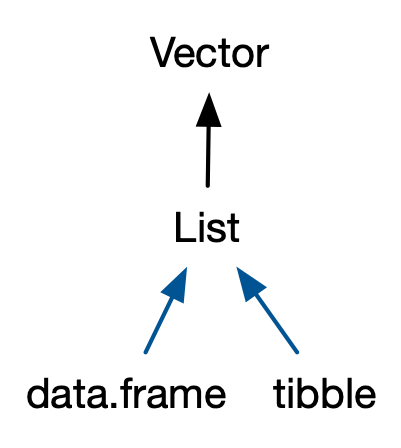] ```{r tbl-type} ``` ] ??? * multi cls: left to right, specific to more general --- ## Why tibble not data frame? .pull-left[ ```{r ref.label = "data-frame"} ``` ] .pull-right[ ```{r eval = FALSE} sci_tbl <- tibble( department = dept, count = nstaff, percentage = count / sum(count)) #<< sci_tbl ``` ```{r ref.label = "tibbles", highlight.output = c(1, 3), echo = FALSE} ``` ] ??? * tibble's display: friendly & informative --- ## Glimpse data ```{r glimpse} ``` Data types and their abbreviations .pull-left[ * `chr`: character * `dbl`: double * `int`: integer * `lgl`: logical ] .pull-right[ * `fct`: factor * `date`: date * `dttm`: date-time * more [column data types](https://tibble.tidyverse.org/articles/types.html) ] ??? text in pink suggest links --- ## Subsetting tibble .left-column[ ### - to 1d ] .right-column[ * with `[[]]` or `\$` ```{r subset-vct} ``` ] --- ## Subsetting tibble .left-column[ ### - to 1d ### - by columns ] .right-column[ * with `[]` or `[, col]` .pull-left[ ```{r subset-col1} ``` ] .pull-right[ ```{r subset-col2} ``` ] ] --- ## Subsetting tibble .left-column[ ### - to 1d ### - by columns ### - by rows ] .right-column[ * with `[row, ]` .pull-left[ ```{r subset-row1} ``` ] .pull-right[ ```{r subset-row2} ``` ] ] --- ## Subsetting tibble .left-column[ ### - to 1d ### - by columns ### - by rows ### - by cols & rows ] .right-column[ * with `[row, col]` ```{r subset-cr, results = "hold", eval = 1} ``` ] --- ## Subsetting tibble * Use `[[` to extract 1d vectors from 2d tibbles * Use `[` to subset tibbles to a new tibble + numbers (positive/negative) as indices + characters (column names) as indices + logicals as indices ```{r ref.label = "subset-cr", eval = FALSE} ``` --- class: middle inverse ## The [tidyverse](https://www.tidyverse.org) is an opinionated [collection of R packages](https://www.tidyverse.org/packages/) designed for data science. *All packages share an underlying design philosophy, grammar, and data structures.* --- ## Use {tidyverse} ```{r tidyverse, message = TRUE, cache = FALSE} library(tidyverse) ``` --- class: inverse middle # Data import `r emo::ji('arrow_down')` --- background-image: url(img/pisa.png) .footnote[] ??? * 3M students from more than 90 countries * conducted every 3 yrs --- .left-column[ .center[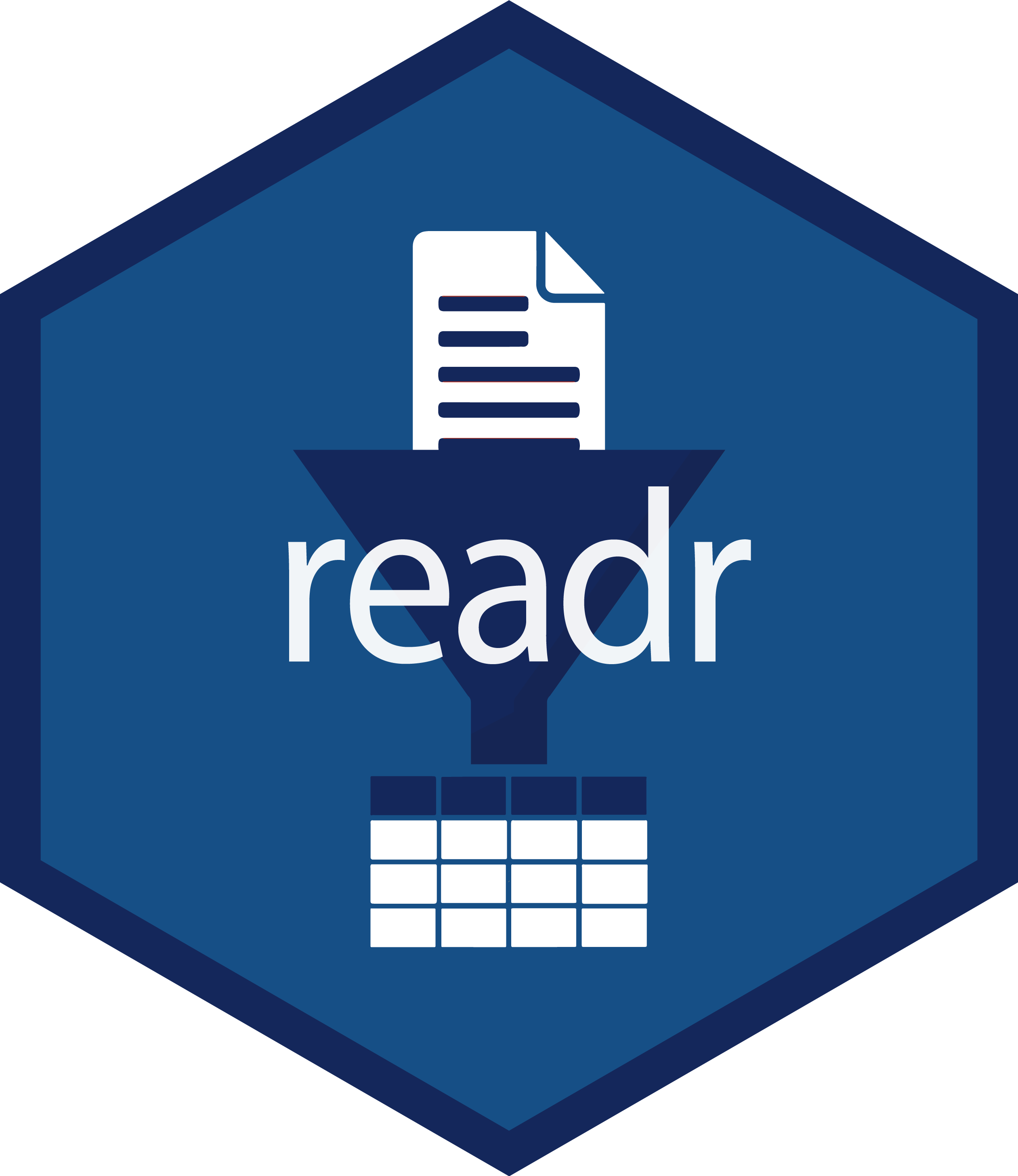] ] .right-column[ ## Reading plain-text rectangular files ### .small[(a.k.a. flat or spreadsheet-like files)] * delimited text files with `read_delim()` + `.csv`: comma separated values with `read_csv()` + `.tsv`: tab separated values `read_tsv()` * `.fwf`: fixed width files with `read_fwf()`
```{bash pisa-header} head -4 data/pisa/pisa-student.csv # shell command, not R ``` ] --- .left-column[ .center[] ] .right-column[ ## Reading comma delimited files ```{r read-csv} ``` ] ??? * from external files in a disk to a tibble obj in R --- ## Let's talk about the file path again! ```{r ref.label = "read-csv", eval = FALSE, echo = 2} ``` `data/pisa/pisa-student.csv` relative to the top-level (or root) directory: * `stats220.Rproj` * `data/` * `pisa/pisa-student.csv` If you don't like `/`, you can use `here::here()` instead. ```{r here, eval = FALSE} read_csv(here::here("data", "pisa", "pisa-student.csv")) ``` .footnote[NOTE: I use the `here()` function from the {here} package using `pkg::fun()`, without calling `library(here)` the ususal way.] --- .left-column[ .center[] ] .right-column[ ## `read_csv()` arguments with [`?read_csv()`](https://readr.tidyverse.org/reference/read_delim.html) ```r read_csv( file, col_names = TRUE, col_types = NULL, locale = default_locale(), na = c("", "NA"), quoted_na = TRUE, quote = "\"", comment = "", trim_ws = TRUE, skip = 0, n_max = Inf, guess_max = min(1000, n_max), progress = show_progress(), skip_empty_rows = TRUE ) ``` ] ??? * w/o using arguments, readr makes smart guesses, which means take a little longer * more specific, speed up the reading --- .left-column[ .center[] ] .right-column[ ## Faster delimited reader at **1.4GB/sec** .center[![](https://github.com/r-lib/vroom/raw/gh-pages/taylor.gif)] ```{r vroom, eval = FALSE} ``` ] ??? * {readr} as toyota, {vroom} sports car * super optimized for fast reading, likely have edge cases, better not for production * when {vroom} moves to a more stable lifecylce, backend {readr} --- .left-column[ .center[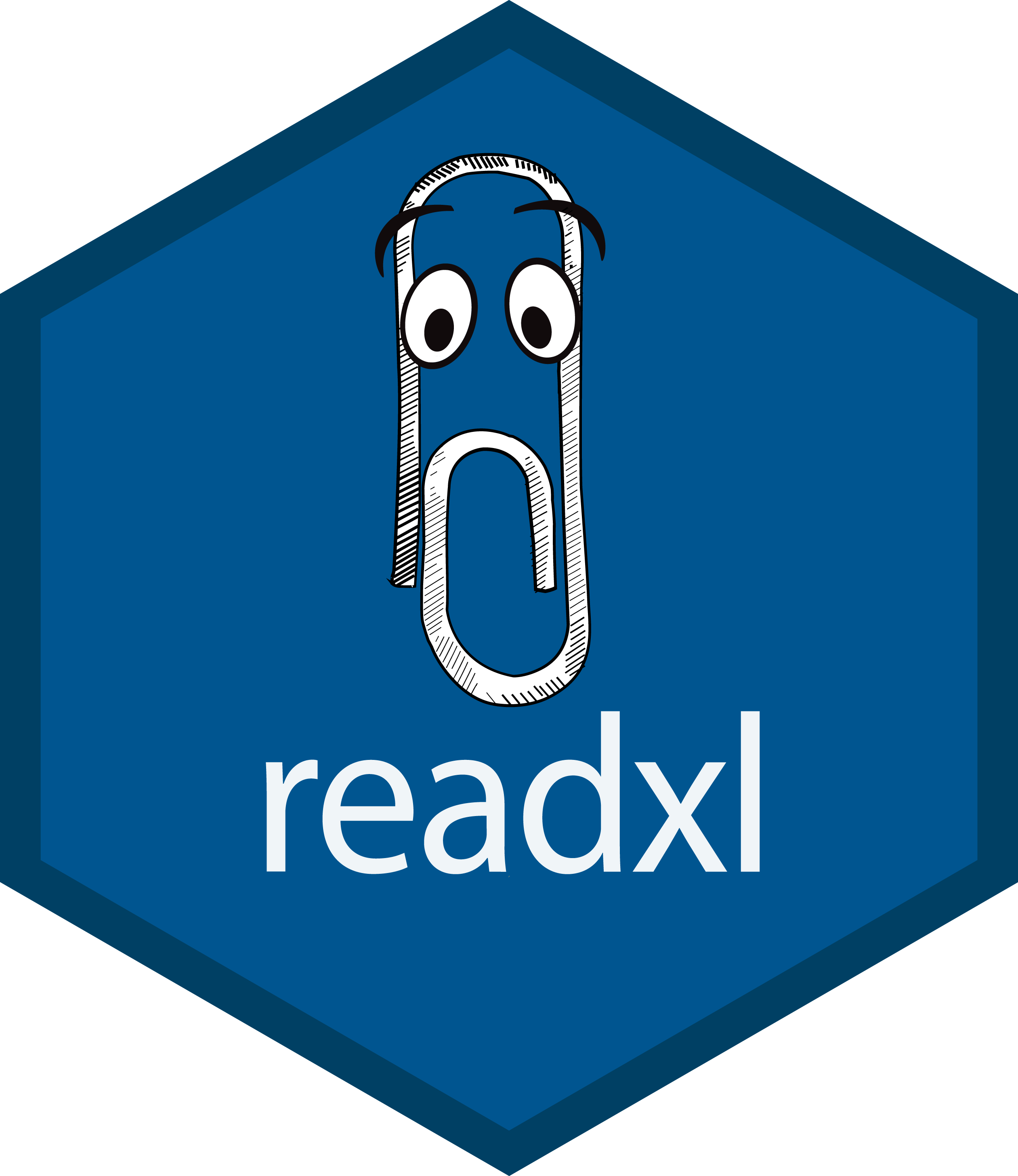] ] .right-column[ ## Reading proprietary binary files * Microsoft Excel + `.xls`: MSFT Excel 2003 and earlier + `.xlsx`: MSFT Excel 2007 and later ```{r excel} ``` ] ??? * contrasting to plain-text, binary files have to be opened by a certain app --- .left-column[ .center[] ] .right-column[ ## Reading proprietary binary files * SAS + `.sas7bdat` with `read_sas()` * Stata + `.dta` with `read_dta()` * SPSS + `.sav` with `read_sav()` ```{r haven, eval = FALSE} ```
Raw PISA data is made available in SAS and SPSS data formats. .footnote[ data source: [https://www.oecd.org/pisa/data/2018database/](https://www.oecd.org/pisa/data/2018database/) ] ] --- class: middle ## Your turn > What is the R data format for a single object? What is its file extension? --- class: middle ## Well, SQL! * **Structured Query Language** for accessing and manipulating databases. * Relational database management systems + [SQLite](https://www.sqlite.org/index.html) + [MySQL](https://www.mysql.com) + PostgresSQL + BigQuery + Spark SQL ### However, 220 is all about R! --- .left-column[ ## {DBI} ] .right-column[ ## Connecting R to database* ```{r database, cache = FALSE} ``` .footnote[NOTE: slides marked with `*` are not examinable.] ] ??? * dbi: database interface, communicating b/t R and db * connecting to SQLite * multi tables typically: students, schools * fields = column names --- .left-column[ ## {DBI} ] .right-column[ ## Connecting R to database* * reading data from database ```{r db-read, eval = FALSE} ``` * writing SQL queries to read chunks ```{r db-query, eval = FALSE} ``` * closing connection ```{r db-close, eval = FALSE} ``` ] --- .left-column[ .center[] ] .right-column[ ## Reading chunks for larger than memory data* ```{r chunked, eval = FALSE} ``` ] ??? * GPU, disk size, RAM * data files in disk * R obj in RAM * crashed, blow up my RAM for reading pisa twice --- .left-column[ ## {jsonlite} ] .right-column[ ## JSON: JavaScript Object Notation * object: `{}` * array: `[]` * value: string/character, number, object, array, logical, `null` .pull-left[ ### JSON ```json { "firstName": "Earo", "lastName": "Wang", "address": { "city": "Auckland", "postalCode": 1010 } "logical": [true, false] } ``` ] .pull-right[ ### R list ```r list( firstName = "Earo", lastName = "Wang", address = list( city = "Auckland", postalCode = 1010 ), logical = c(TRUE, FALSE) ) ``` ] ] ??? * a lightweight text format * easy for humans to read and write * easy for machines to parse and generate * annologue to list * `null` is `NA` --- .left-column[ ## {jsonlite} ] .right-column[ ## Reading json files ```{r json} ``` ] ??? * read from url * but url is temporary * labs/assignments must use relative path, no web url accepted --- .left-column[ ## {jsonlite} ] .right-column[ ## Reading json files as tibbles ```{r json-df} ``` ] --- .left-column[ .center[] ] .right-column[ ## Reading spatial data* ```{r sf} ``` .footnote[data source: [**Auckland Transport Open GIS Data**](https://data-atgis.opendata.arcgis.com/datasets/bus-route/data?geometry=169.841%2C-37.610%2C179.685%2C-36.072)] ] ??? * sf: simple features * spatial data: points, lines from a to b (bus routes), polygons --- .left-column[ .center[] ] .right-column[ ## Reading spatial data* ```{r sf, message = -1} ``` .footnote[data source: [**Auckland Transport Open GIS Data**](https://data-atgis.opendata.arcgis.com/datasets/bus-route/data?geometry=169.841%2C-37.610%2C179.685%2C-36.072)] ] --- .left-column[ .center[] ] .right-column[ ## Reading spatial data* ```{r sf-print} ``` ] --- .left-column[ .center[] ] .right-column[ ## Spatial visualisation* .panelset[ .panel[.panel-name[Map] ```{r sf-plot, out.width = "78%", echo = FALSE} ``` .panel[.panel-name[R Code] ```{r ref.label = "sf-plot", eval = FALSE} ``` ] ] ] ] ??? * rich data fmts: audio, images, etc * seen an unseen file type: google that type and the corresponding r function --- class: inverse middle # Data export `r emo::ji('arrow_up')` --- class: middle ## From `read_*()` to `write_*()` ```{r write-movies, eval = FALSE} ``` --- ## Reading .pull-left[ .center[[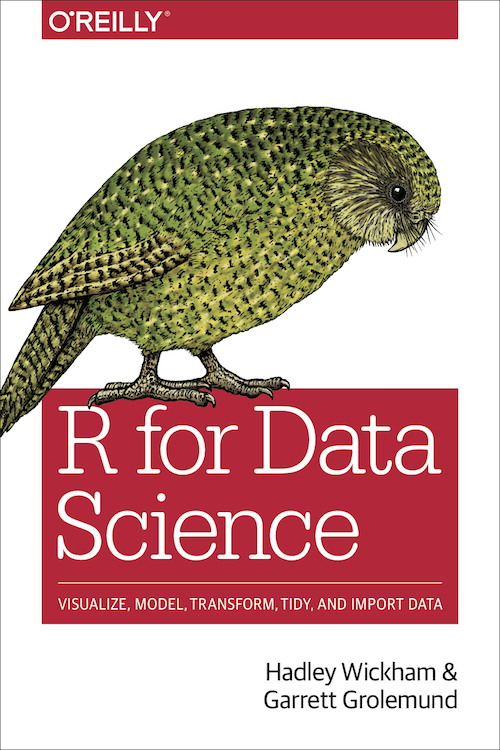](https://r4ds.had.co.nz)] * [Tibbles](https://r4ds.had.co.nz/tibbles.html) * [Data import](https://r4ds.had.co.nz/data-import.html) ] .pull-right[ .center[[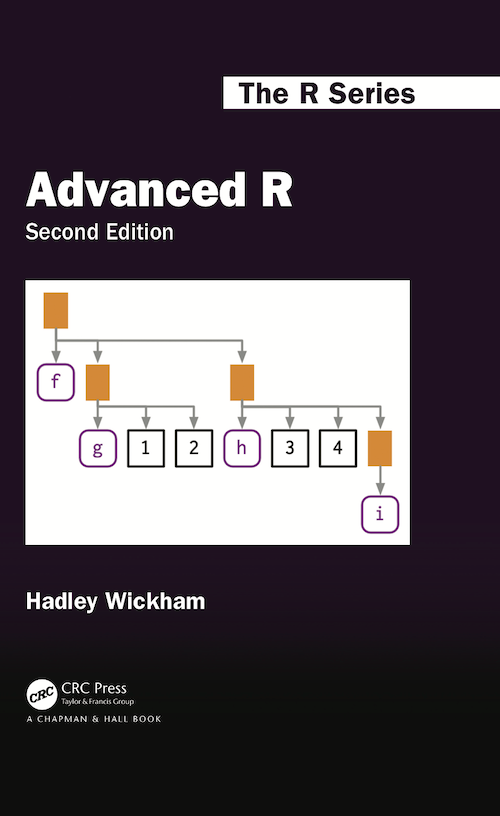](https://adv-r.hadley.nz)] * [Subsetting](https://adv-r.hadley.nz/subsetting.html#subset-single) ]